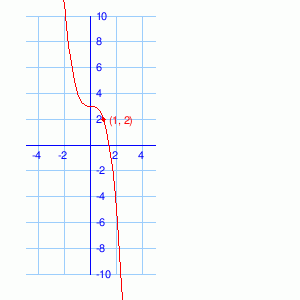# Math Practice Topic: Nonlinear Functions

## Sample Levels (out of 3)

Give the equation of the following functions. They may be quadratic or cubic functions. They should be of the form 'y = nx2 + b' or 'y = nx3 + b' where n and b are integers.

 1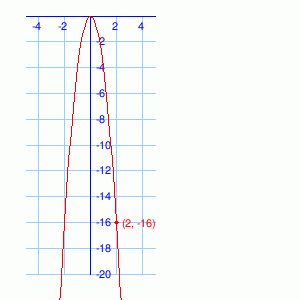2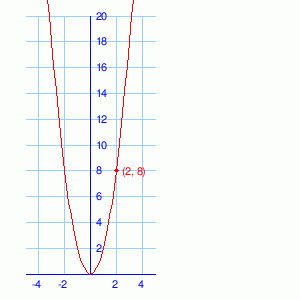Give the equation of the following functions. They may be quadratic or cubic functions. They should be of the form 'y = nx2 + b' or 'y = nx3 + b' where n and b are integers.

 1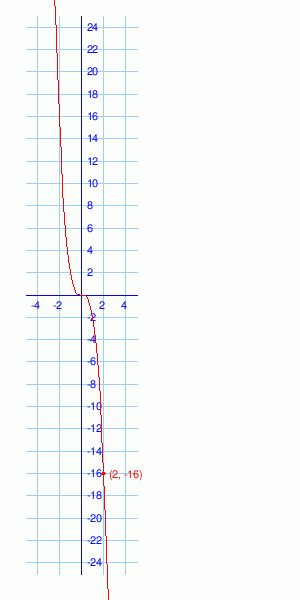2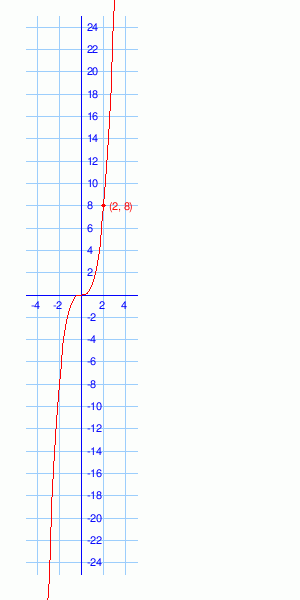Give the equation of the following functions. They may be quadratic or cubic functions. They should be of the form 'y = nx2 + b' or 'y = nx3 + b' where n and b are integers.

 1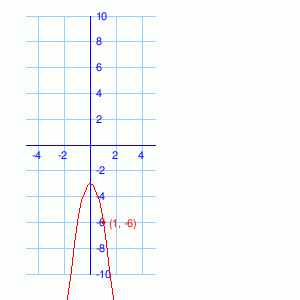2